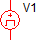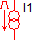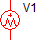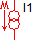# 2.1.1 The Initial Conditions (.INIT) File

SIMPLIS creates an initial conditions (.INIT) file at the end of every SIMPLIS simulation. This plain text file contains an initial condition statement for every inductor current, capacitor voltage, switch state, and logic device state. The file has several uses, which you will learn both in this topic and the next topic, 2.1.2 Back Annotating a Schematic.

In this topic:

## Key Concepts

This topic addresses the following key concepts:

• SIMPLIS saves the final state of the circuit after each simulation completes to a text file called the .INIT file.
• You can use the .INIT file to initialize the circuit to a known state.
• Unlike the snapshot loading, which is automatic, you have to tell the simulator to use information in the .INIT file.
• The state of large signal sources, such as sinusoidal voltage or current sources, as well as PWL sources are not included in the .INIT file. The state of these sources is captured in a Snapshot.

## What You Will Learn

In this topic, you will learn the following:

• How to view or edit the .INIT file.
• The difference between Snapshots and the .INIT file.

## Getting Started

### Exercise #1: Viewing the .INIT File

1. Open the schematic 2.2_PFC_Critical_Conduction_Mode.sxsch.
2. Press F9 to run the simulation.
3. From the menu bar, select Simulator > Initial Conditions > Edit .INIT File .
Result: The initial conditions file 2.2_PFC_Critical_Conduction_Mode.deck.init opens in a text editor window. The text is shown below:
```*
*    Tue Sep 29 13:16:14 2015
*
.INIT	X\$Q1.X1_BASE.QQ1	=	 OPEN
.INIT	X\$S3.S1	=	 OPEN
.INIT	X\$S4.S1	=	 OPEN
.INIT	X\$U1.X\$S2.S1	=	 OPEN
.INIT	X\$U1.X\$S3.S1	=	 CLOSE
.INIT	X\$U1.X\$U11.SW_BOT	=	 CLOSE
.INIT	X\$U1.X\$U11.SW_TOP	=	 OPEN
.INIT	!R\$R1	=	 1
.INIT	X\$D1.X_BASE.!R_D_POWER	=	 1
.INIT	X2.!R_SLEW_RATE	=	 2
.INIT	X2.!R_VM_LIMIT	=	 1
.INIT	X2.!R_VP_LIMIT	=	 1
.INIT	X\$D10.X_BASE_AC1_PLUS.!R_D_POWER	=	 1
.INIT	X\$D10.X_BASE_AC2_PLUS.!R_D_POWER	=	 1
.INIT	X\$D10.X_BASE_MINUS_AC1.!R_D_POWER	=	 1
.INIT	X\$D10.X_BASE_MINUS_AC2.!R_D_POWER	=	 1
.INIT	X\$Q1.X1_BASE.!R_DBODY	=	 3
.INIT	X2.!R_FF2	=	 2
.INIT	X2.!R_FF3	=	 1
.INIT	X2.!R_IOUT_LIMIT	=	 2
.INIT	X\$U1.X\$U11.X\$U_ZERO_DELAY.!DCOMP	=	 0
.INIT	X\$U1.X\$U1.A_OR:IC	=	 0
.INIT	X\$U1.X\$U10.A_SRLATCH:IC	=	 0
.INIT	X\$U1.X\$U6.A_OR:IC	=	 0
.INIT	X\$U1.X\$U8.A_COMP:IC	=	 1
.INIT	X\$U1.X\$U12.A_AND:IC	=	 0
.INIT	X\$U1.X\$U13.A_BUF:IC	=	 0
.INIT	X\$U1.X\$U14.A_COMP:IC	=	 0
.INIT	X\$U1.X\$U15.A_SRLATCH:IC	=	 0
.INIT	X\$U1.X\$U2.A_SRLATCH:IC	=	 1
.INIT	X\$U1.X\$U3.A_AND:IC	=	 0
.INIT	X\$U1.X\$U4.A_COMP:IC	=	 0
.INIT	X\$U1.X\$U5.A_DFF:IC	=	 1
.INIT	V(C10)	=	-3.985749308422935e-001
.INIT	V(C2)	=	-6.732810161586880e-001
.INIT	V(X\$Q1.X1_BASE.X\$CGS.C_SINGLE_SEGMENT)	=	2.979998541403869e-006
.INIT	V(X\$U1.C1)	=	1.580901973288782e+000
.INIT	V(X\$U1.C2)	=	-1.999999800000020e-009
.INIT	V(X2.C2)	=	-6.288549723703802e+000
.INIT	V(C3)	=	-2.275557668440759e-001
.INIT	V(C5)	=	1.438485157912415e+000
.INIT	V(C6)	=	1.958034002604594e+001
.INIT	V(C7)	=	-7.607413581071337e-002
.INIT	V(C8)	=	-7.978167763421369e-001
.INIT	V(C9)	=	-3.985756142680252e-001
.INIT	V(X\$C1.CAP)	=	1.887415500717153e+002
.INIT	V(X\$Q1.X1_BASE.X\$CDS.C_SINGLE_SEGMENT)	=	-7.023271322771595e-001
.INIT	I(X\$L1.L_IND)	=	-2.740344407105104e-002
.INIT	I(X\$L2.L_IND)	=	-7.180619014816601e-002
.INIT	I(X\$L3.L_IND)	=	2.231814660546221e-002
.INIT	I(X\$L4.L_IND)	=	-2.231814660546221e-002
```

## Discussion

After every POP or Transient simulation, SIMPLIS generates a text file that records the state of the system at the last time point of the simulation. The text file is located in the SIMPLIS_Data directory and has the filename <schematic_name>.deck.init, where <schematic_name> is the name of the schematic from which the .INIT file was generated. The .INIT file contains the last operating point of:

• All SIMPLIS primitive switches. Each extracted SIMPLIS MOSFET model includes one of these switches.
• The operating PWL segment of all PWL resistors.
• The logic state of all digital logic.
• The current through each inductor and the voltage across each capacitor, including PWL inductors and capacitors.

As you can see, the .INIT file contains a very comprehensive state of the circuit at the last simulation time point. You can therefore utilize this information to initialize a schematic to this last simulation state. In the SIMetrix/SIMPLIS environment, this operation is called Back Annotation. Back annotation takes the final conditions of one simulation and back annotates these final conditions to be the initial conditions of the next simulation for virtually all the components in a SIMPLIS schematic. Initial conditions mean the initial conditions of each component at t = 0 of a subsequent simulation. Back annotation forces the final conditions of one simulation to be the initial t = 0 conditions of the next simulation.

There are only two devices whose final state is excluded from the .INIT file,

• The large signal time dependent sources, such as sinusoidal voltage or current sources.
• PWL voltage and current sources.

These independent sources use the waveform generator and PWL source symbols, shown below.The values of these independent sources at t = 0 are already defined by the user. There is no meaningful way to resolve the conflict between the source definitions set up by the user and an arbitrary set of "initial conditions" resulting from the back annotation process. As a result, users need to be keenly aware that these sources cannot be back annotated. Lack of awareness of this point can lead to critical modeling flaws and the negative follow-on impacts cannot be overstated. A model which cannot back annotate properly often will not POP, and therefore an AC analysis cannot be performed on the circuit. Often these models require the output voltage to start from zero every simulation, which greatly increases the time required to get to the converter to steady-state.

### Differences Between Snapshots and .INIT Files

A natural question arises - "What are the differences between a Snapshot and a .INIT file?" In section 2.0 Transient Analysis Settings, you learned about transient snapshots. The .INIT file is different than a snapshot in several ways:

• The state of the large signal sources, such as the Sine wave source V1 in the 2.2_PFC_Critical_Conduction_Mode.sxsch schematic, are NOT captured in a .INIT file, but ARE captured in a snapshot.
• You can edit an .INIT file for your own purposes, or even generate a .INIT file from scratch. The snapshot information is saved in a syntax only readable by SIMPLIS.

In the next section 2.1.2 Back Annotating a Schematic, you will learn several ways to back annotate the schematic.

## Conclusions and Key Points to Remember

• The .INIT file is a key mechanism used in SIMPLIS to save the state of the simulation.
• The state of the large signal independent sources, including PWL sources used as ramp generators or as initialization pulse sources are not captured in the .INIT file, but are captured in a Snapshot.